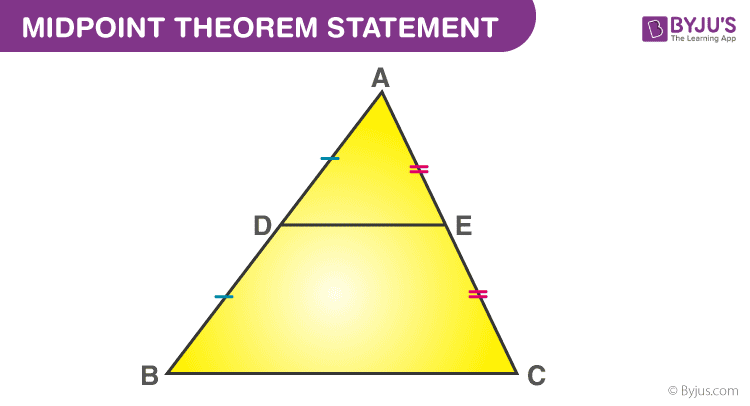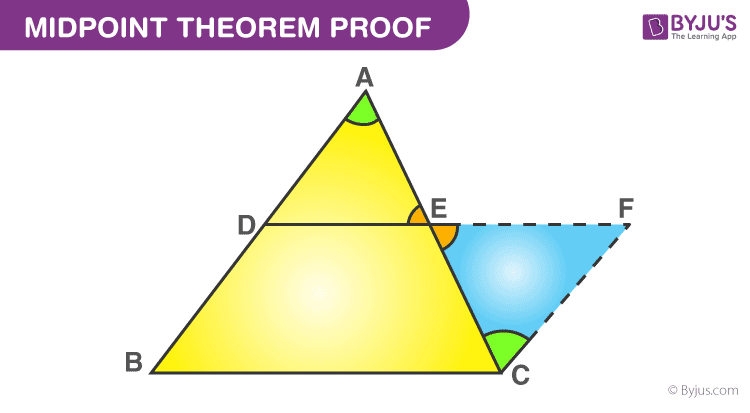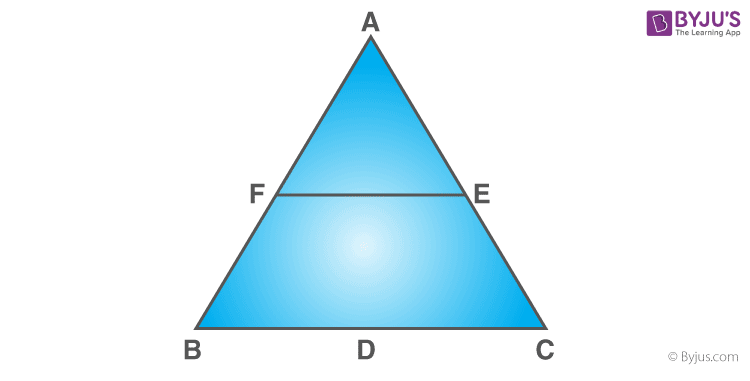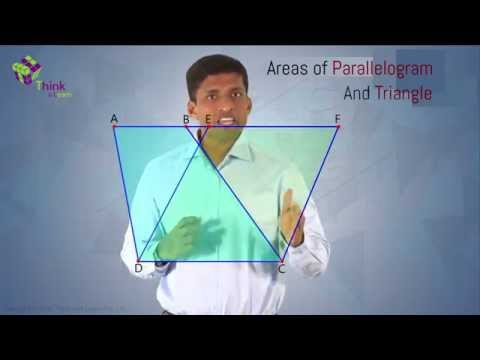# Mid Point Theorem

Geometry is one of the significant and essential branches of mathematics. This field deals with the geometrical problems and figures which are based on their properties. One of the important theorems in the field of geometry that deals with the properties of triangles are called the Mid- Point Theorem.

The theory of midpoint theorem is used in coordinate geometry, stating that the midpoint of the line segment is an average of the endpoints. The ‘x’ and the ‘y’ coordinates must be known for solving an equation using this theorem. The Mid- Point Theorem is also useful in the fields of calculus and algebra.

## Mid-Point Theorem Statement

The midpoint theorem states that “The line segment in a triangle joining the midpoint of any two sides of the triangle is said to be parallel to its third side and is also half of the length of the third side.”## Mid-Point Theorem Proof

If a line segment adjoins the mid-point of any two sides of a triangle, then the line segment is said to be parallel to the remaining third side and its measure will be half of the third side.

Consider the triangle ABC, as shown in the above figure,

Let E and D be the midpoints of the sides AC and AB. Then the line DE is said to be parallel to the side BC, whereas the side DE is half of the side BC; i.e., DE || BC

DE = (1/2 *  BC).

Now consider the below figure,Construction-  Extend the line segment DE and produce it to F such that, EF = DE and join CF.

EC = AE —–   (given)

∠CEF = ∠AED (vertically opposite angles)

DE = EF ( By construction )

By SAS congruence criterion,

Therefore,

∠FCE= ∠DAE    {by c.p.c.t.}

and CF = AD {by c.p.c.t.}

∠CFE and ∠ADE are the alternate interior angles.

Assume CF and AB as two lines which are intersected by the transversal DF.

In a similar way, ∠FCE and ∠DAE are the alternate interior angles.

Assume CF and AB are the two lines which are intersected by the transversal AC.

Therefore, CF ∥ AB

So, CF ∥ BD

and CF = BD  {since BD = AD, it is proved that CF = AD}

Thus, BDFC forms a parallelogram.

By the properties of a parallelogram, we can write

BC ∥ DF

and BC = DF

BC ∥ DE

and DE = (1/2 *  BC).

Hence, the midpoint theorem is proved.

## Mid-Point Theorem Formula

In Coordinate Geometry, the midpoint theorem refers to the midpoint of the line segment. It defines the coordinate points of the midpoint of the line segment and can be found by taking the average of the coordinates of the given endpoints. The midpoint formula is used to determine the midpoint between the two given points.

If P1(x1, y1) and P2(x2, y2) are the coordinates of two given endpoints, then the midpoint formula is given as:

Midpoint = [(x+ x2)/2, (y+ y2)/2]

### The converse of Mid-Point Theorem

The converse of the midpoint theorem states that ” if a line is drawn through the midpoint of one side of a triangle, and parallel to the other side, it bisects the third side”.

### Midpoint Theorem Example

The example is given below to understand the midpoint theorem.

Example:

In triangle ABC, the midpoints of BC, CA, and AB are D, E, and F, respectively. Find the value of EF, if the value of BC = 14 cmSolution:

Given: BC = 14 cm

If F is the midpoint of AB and E is the midpoint of AC, then using the midpoint theorem:

EF = 1/2 (BC)

Substituting the value of BC,

EF = (1/2) × 14

EF = 7 cm

Therefore, the value of EF = 7cm.The Mid-Point Theorem can also be proved using triangles. Suppose two lines are drawn parallel to the x and the y-axis, which begin at endpoints and are connected through the midpoint, then the segment passes through the angle between them, resulting in two similar triangles. This relation of these triangles forms the Mid- Point Theorem.

## Frequently Asked Questions – FAQs

Q1

### What does the midpoint theorem state?

The midpoint theorem states that “The line segment in a triangle joining the midpoint of two sides of the triangle is said to be parallel to its third side and is also half of the length of the third side.”
Q2

### What is the midpoint theorem and converse of the Midpoint Theorem?

The midpoint theorem states that “The line segment in a triangle joining the midpoint of two sides of the triangle is said to be parallel to its third side and is also half of the length of the third side.”
Converse: The line drawn through the midpoint of one side of a triangle, parallel to another side bisects the third side.
Q3

### What is the definition of midpoint in proofs?

In geometry, the midpoint is defined as the point which divides the line segment into two equal parts.
Q4

### What does midpoint mean?

Midpoint means a point which lies at or near the middle or equidistant from both ends of the line segment. In other words, the midpoint is the position of a fact which is halfway between the beginning and the end.

1. Very nice

2. Very good

3. Thanks for the information and it is very useful for students like us.

4. this is so amazing because tommorow is my maths exam halfy yearly .very good keep it up

5. it was very much helpfull

6. That was clear:)

7. thanx very usefull

8. Thanks , I understood it easily .